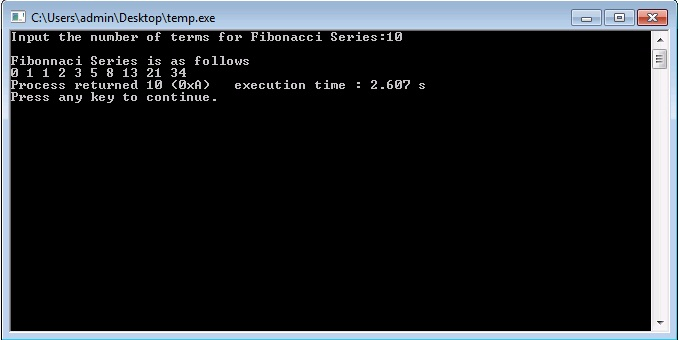# C/C++ Program for Fibonacci Series Using Recursion

Series 0, 1, 1, 2, 3, 5, 8, 13, 21 . . . . . . . is a Fibonacci series. In Fibonacci series, each term is the sum of the two preceding terms.

The C and C++ program for Fibonacci series using recursion is given below.

### C++ Program

Output:### 18 thoughts on “C/C++ Program for Fibonacci Series Using Recursion”

1.i guess 0 should not have been a part of the series….
so in the function u should have used return fibbonacci(n)+fibbonacci(n-1)
please correct me if i am wrong

1.For not getting 0 in the output,
i can be initialized as 1 instead of 0 : int i=1;

1.Write a recursive program for implementing a Fibonacci of any
number

2.The while statement needs to be, while(i <= n)(line 24), and (int i = 0) needs to be initialized at 1(line 19), not at 0. Changing this will result in the proper value for any fibonacci(n). As is right now, it is giving you the value at fibonacci(n-1), hence the reason fibonacci(8) yields a value of 13.

3.Can someone help me with this, write a recursive program to implement the Fibonacci series from 0 to 21.

1.#include

using namespace std;

int Fib(int n);

int main() {
int i;

for(i=1;i<=20;i++) {
cout << "Fib(" << i << ") = " << Fib(i) << endl;
}

return 0;
}

int Fib(int n) {
int returnValue;

// handle the base case first;
if (n == 1 or n == 2) {
returnValue = 1;
} else {
returnValue = Fib(n-1) + Fib(n-2);
}
return returnValue;
}

1.Can you help me with this, write
a recursive program to implement the
Fibonacci series a given number?

1.#include

using namespace std;

int Fib(int n);

int main() {
int i;

for(i=1;i<=20;i++) {
cout << "Fib(" << i << ") = " << Fib(i) << endl;
}

return 0;
}

int Fib(int n) {
int returnValue;

// handle the base case first;
if (n == 1 or n == 2) {
returnValue = 1;
} else {
returnValue = Fib(n-1) + Fib(n-2);
}
return returnValue;
}

4.sm. shawon srower

thanks

5.so nice ans

6.i relly appreciet you!!!!!!!!!!!!!!!!!!!!!!!!!!!!!!!!!!!!!!!

7.i need to get the output as, if i enter 15 as input then i should get the answer as 0,1,1,2,3,5,8,13.so, where should i have to change the code?

8.hi. if we want just the last number of this, what should we do ?
we give : 3
we get : 2

1.9.i dont know programming

10.I love books!! <3 xbbb hehehehe!!

11.Not actual Recursion. Its while loop which does the required part and not the method. Good to move the logic inside the method

void Recursion::FibonaciiSeries(int num)
{
static int print = 1;
static int next = 2;

if (num == 0)
{
return;
}
else
{
cout << print << " ";
int temp = print;
print = next;
next = print + temp;
FibonaciiSeries(num – 1);
}
}

12.if you put 50 that is not work. could you check am i right?